cancel
Showing results for
Did you mean:Frequent Visitor

## Dynamic Visual / Is there a way, to use a user Input in dax calculation.

Actual Problem:

I just make a column Visual to display Monthly values, and a calculated Value for the previous year and the the Year to day.

For the Visual i build a calculated tabel like this: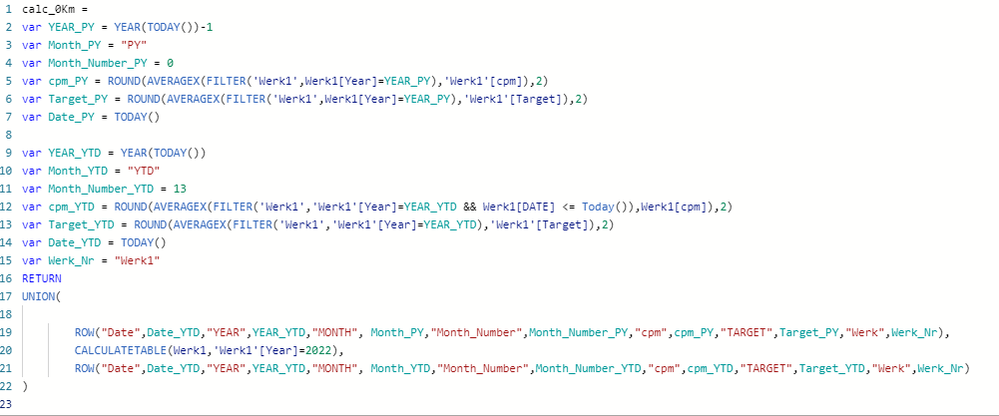DAX CODE

The visual looks like this if i chosse Werk1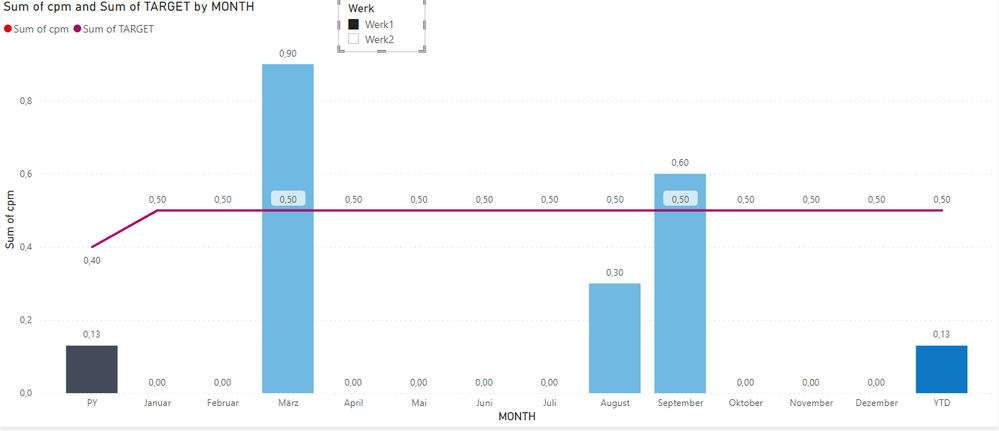Now i want to use a filter for different Plants for the user of the Dashboard. Have anyone an idea hot to get a User input into a Variable to use the value in the calculated Table

If use a slicer the PY and YTD column disappears if i choose Werk2.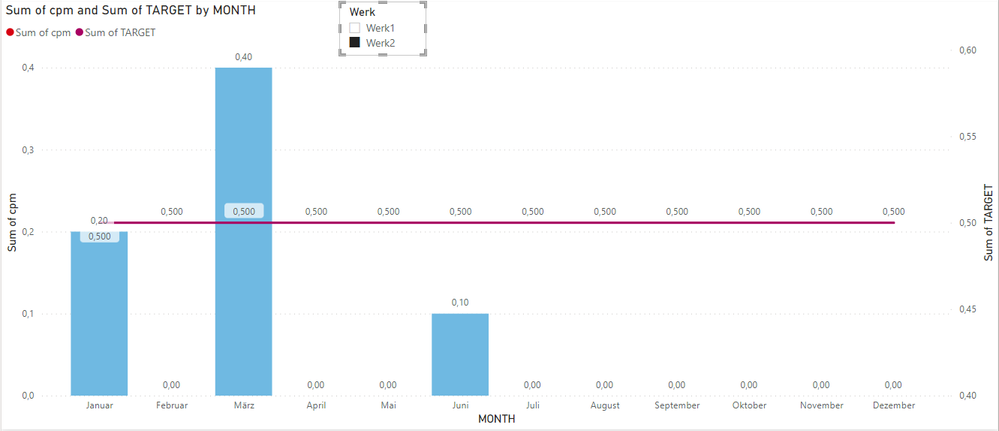Does anyone know a way to get the user input into the DAX code?

2 ACCEPTED SOLUTIONSCommunity Support

In my test, there is only "Werk1" in your calculated table: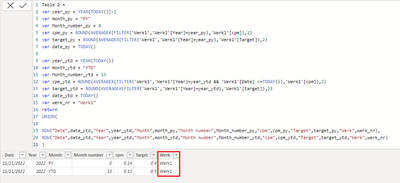Please try following DAX ti create a new table:

``````Table =
var year_py_1 = YEAR(TODAY())-1
var month_py_1 = "PY"
var Month_number_py_1 = 0
var cpm_py_1 = ROUND(AVERAGEX(FILTER('Werk1','Werk1'[Year]=year_py_1 && 'Werk1'[Plant] = "Werk1"),'Werk1'[cpm]),2)
var target_py_1 = ROUND(AVERAGEX(FILTER('Werk1','Werk1'[Year]=year_py_1 && 'Werk1'[Plant] = "Werk1"),'Werk1'[Target]),2)
var date_py_1 = TODAY()

var year_ytd_1 = YEAR(TODAY())
var month_ytd_1 = "YTD"
var Month_number_ytd_1 = 13
var cpm_ytd_1 = ROUND(AVERAGEX(FILTER('Werk1','Werk1'[Year]=year_ytd_1 && 'Werk1'[Date] <=TODAY() && 'Werk1'[Plant] = "Werk1"),'Werk1'[cpm]),2)
var target_ytd_1 = ROUND(AVERAGEX(FILTER('Werk1','Werk1'[Year]=year_ytd_1 && 'Werk1'[Plant] = "Werk1"),'Werk1'[target]),2)
var date_ytd_1 = TODAY()
var werk_nr_1 = "Werk1"

var year_py_2 = YEAR(TODAY())-1
var month_py_2 = "PY"
var Month_number_py_2 = 0
var cpm_py_2 = ROUND(AVERAGEX(FILTER('Werk1','Werk1'[Year]=year_py_2 && 'Werk1'[Plant] = "Werk2"),'Werk1'[cpm]),2)
var target_py_2 = ROUND(AVERAGEX(FILTER('Werk1','Werk1'[Year]=year_py_2 && 'Werk1'[Plant] = "Werk2"),'Werk1'[Target]),2)
var date_py_2 = TODAY()

var year_ytd_2 = YEAR(TODAY())
var month_ytd_2 = "YTD"
var Month_number_ytd_2 = 13
var cpm_ytd_2 = ROUND(AVERAGEX(FILTER('Werk1','Werk1'[Year]=year_ytd_2 && 'Werk1'[Date] <=TODAY() && 'Werk1'[Plant] = "Werk2"),'Werk1'[cpm]),2)
var target_ytd_2 = ROUND(AVERAGEX(FILTER('Werk1','Werk1'[Year]=year_ytd_2 && 'Werk1'[Plant] = "Werk2"),'Werk1'[target]),2)
var date_ytd_2 = TODAY()
var werk_nr_2 = "Werk2"

return
UNION(

ROW("Date",date_py_1,"Year",year_py_1,"Month",month_py_1,"Month number",Month_number_py_1,"cpm",cpm_py_1,"Target",target_py_1,"Plant",werk_nr_1),
ROW("Date",date_ytd_1,"Year",year_ytd_1,"Month",month_ytd_1,"Month number",Month_number_ytd_1,"cpm",cpm_ytd_1,"Target",target_ytd_1,"Plant",werk_nr_1),
ROW("Date",date_py_2,"Year",year_py_2,"Month",month_py_2,"Month number",Month_number_py_2,"cpm",cpm_py_2,"Target",target_py_2,"Plant",werk_nr_2),
ROW("Date",date_ytd_2,"Year",year_ytd_2,"Month",month_ytd_2,"Month number",Month_number_ytd_2,"cpm",cpm_ytd_2,"Target",target_ytd_2,"Plant",werk_nr_2)
)``````

You will get a atable like this: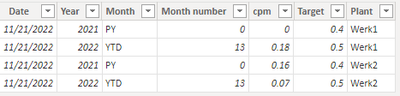Union two tables:

``````NewTable = UNION(
SELECTCOLUMNS('Table',"Date",'Table'[Date],"cpm",'Table'[cpm],"Target",'Table'[Target],"Plant",'Table'[Plant],"Year",'Table'[Year],"Month",'Table'[Month],"Month number",'Table'[Month number]),
SELECTCOLUMNS('Werk1',"Date",'Werk1'[Date],"cpm",'Werk1'[cpm],"Target",'Werk1'[Target],"Plant",'Werk1'[Plant],"Year",'Werk1'[Year],"Month",'Werk1'[Month],"Month number",'Werk1'[Month number])
)``````You will get the result you want: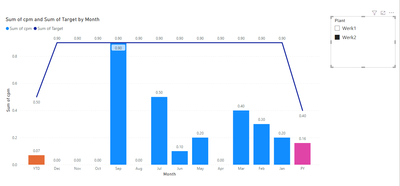Best regards,

If this post helps, then please consider Accept it as the solution to help the other members find it more quickly.Frequent Visitor

I have found my own way to visualize the charts.
I just programmed a Python plot and put it into the Power BI Python-visual.

4 REPLIES 4Frequent Visitor

I have found my own way to visualize the charts.
I just programmed a Python plot and put it into the Power BI Python-visual.Community Support

In my test, there is only "Werk1" in your calculated table:Please try following DAX ti create a new table:

``````Table =
var year_py_1 = YEAR(TODAY())-1
var month_py_1 = "PY"
var Month_number_py_1 = 0
var cpm_py_1 = ROUND(AVERAGEX(FILTER('Werk1','Werk1'[Year]=year_py_1 && 'Werk1'[Plant] = "Werk1"),'Werk1'[cpm]),2)
var target_py_1 = ROUND(AVERAGEX(FILTER('Werk1','Werk1'[Year]=year_py_1 && 'Werk1'[Plant] = "Werk1"),'Werk1'[Target]),2)
var date_py_1 = TODAY()

var year_ytd_1 = YEAR(TODAY())
var month_ytd_1 = "YTD"
var Month_number_ytd_1 = 13
var cpm_ytd_1 = ROUND(AVERAGEX(FILTER('Werk1','Werk1'[Year]=year_ytd_1 && 'Werk1'[Date] <=TODAY() && 'Werk1'[Plant] = "Werk1"),'Werk1'[cpm]),2)
var target_ytd_1 = ROUND(AVERAGEX(FILTER('Werk1','Werk1'[Year]=year_ytd_1 && 'Werk1'[Plant] = "Werk1"),'Werk1'[target]),2)
var date_ytd_1 = TODAY()
var werk_nr_1 = "Werk1"

var year_py_2 = YEAR(TODAY())-1
var month_py_2 = "PY"
var Month_number_py_2 = 0
var cpm_py_2 = ROUND(AVERAGEX(FILTER('Werk1','Werk1'[Year]=year_py_2 && 'Werk1'[Plant] = "Werk2"),'Werk1'[cpm]),2)
var target_py_2 = ROUND(AVERAGEX(FILTER('Werk1','Werk1'[Year]=year_py_2 && 'Werk1'[Plant] = "Werk2"),'Werk1'[Target]),2)
var date_py_2 = TODAY()

var year_ytd_2 = YEAR(TODAY())
var month_ytd_2 = "YTD"
var Month_number_ytd_2 = 13
var cpm_ytd_2 = ROUND(AVERAGEX(FILTER('Werk1','Werk1'[Year]=year_ytd_2 && 'Werk1'[Date] <=TODAY() && 'Werk1'[Plant] = "Werk2"),'Werk1'[cpm]),2)
var target_ytd_2 = ROUND(AVERAGEX(FILTER('Werk1','Werk1'[Year]=year_ytd_2 && 'Werk1'[Plant] = "Werk2"),'Werk1'[target]),2)
var date_ytd_2 = TODAY()
var werk_nr_2 = "Werk2"

return
UNION(

ROW("Date",date_py_1,"Year",year_py_1,"Month",month_py_1,"Month number",Month_number_py_1,"cpm",cpm_py_1,"Target",target_py_1,"Plant",werk_nr_1),
ROW("Date",date_ytd_1,"Year",year_ytd_1,"Month",month_ytd_1,"Month number",Month_number_ytd_1,"cpm",cpm_ytd_1,"Target",target_ytd_1,"Plant",werk_nr_1),
ROW("Date",date_py_2,"Year",year_py_2,"Month",month_py_2,"Month number",Month_number_py_2,"cpm",cpm_py_2,"Target",target_py_2,"Plant",werk_nr_2),
ROW("Date",date_ytd_2,"Year",year_ytd_2,"Month",month_ytd_2,"Month number",Month_number_ytd_2,"cpm",cpm_ytd_2,"Target",target_ytd_2,"Plant",werk_nr_2)
)``````

You will get a atable like this:Union two tables:

``````NewTable = UNION(
SELECTCOLUMNS('Table',"Date",'Table'[Date],"cpm",'Table'[cpm],"Target",'Table'[Target],"Plant",'Table'[Plant],"Year",'Table'[Year],"Month",'Table'[Month],"Month number",'Table'[Month number]),
SELECTCOLUMNS('Werk1',"Date",'Werk1'[Date],"cpm",'Werk1'[cpm],"Target",'Werk1'[Target],"Plant",'Werk1'[Plant],"Year",'Werk1'[Year],"Month",'Werk1'[Month],"Month number",'Werk1'[Month number])
)``````You will get the result you want:Best regards,

If this post helps, then please consider Accept it as the solution to help the other members find it more quickly.Frequent Visitor

The raw data looks like this at the moment.The expected output should look like this for example two plants, in the final version there are plenty more plants.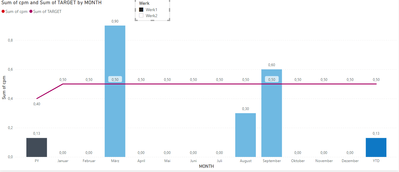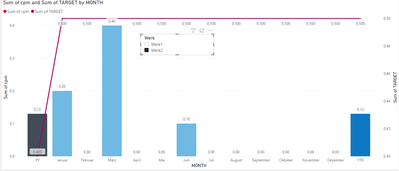I need to calculate the previous year (PY) value and the year today (YT) from the raw data and display it in the column chart with the values from each month.

The user of the dashborad should choose a plant from a filter/slicer and get the visual for the choosen plant

I hope this helps.

Thanks for your help and time.Community Support

In order to better understanding your demands and give the right solution, could you please share with me some screenshots of your data after hiding sensitive information and tell me what's your expected output?

Best regards,